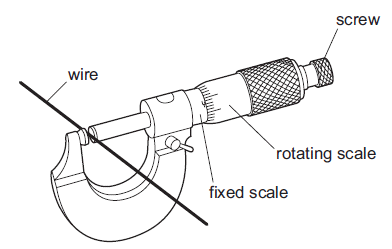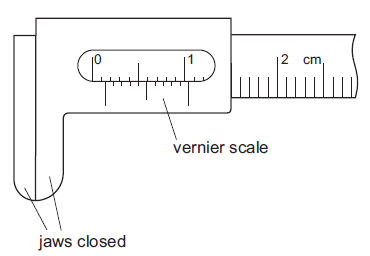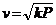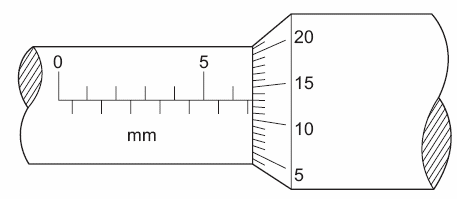PHYSICS FORM 2

ONLINE TESTS SERIES

MEASUREMENTS 2

1. A micrometer is used to measure the diameter of a uniform wire.What is done to obtain an accurate answer?

2. Vernier calipers are shown with the jaws closed.What is the zero error?

1. The variation with speed v of the force F acting on an object is given by the expression

F = pv2 + qv,

where p and q are constants.

What quantity should be plotted on the y-axis of a graph and what should be plotted on the x-axis in order to give a straight-line graph?

 y-axis x-axis A. v B. v2 C. F v D. F v2

1. The speed of sound v in a gas is related to the pressure P of the gas by the expressionwhere k is a constant.

Which variables should be plotted in order to produce a straight-line graph with the slope equal to k?

1. Sub-multiples of units may be expressed using a prefix. Which one of the following lists the prefixes in decreasing order of magnitude?
 A. centi- micro- milli- nano- B. milli- centi- nano- micro- C. centi- milli- micro- nano- D. milli- micro- centi- nano-

1. What is the reading on this micrometer?7 How can you locate the centre of mass of an irregular object?

 8 What is the frequency of a simple pendulum if the time period is ten seconds? (1Hz = 1cycle per second)

 9 Where is the centre of mass located on a rectangle of length 5cm and width 3cm if the mass is distributed evenly throughout the object?

 10 A simple pendulum oscillates 50 times per second. What is the frequency of the pendulum?   (Frequency = cycles per second)

Question 1 of 10